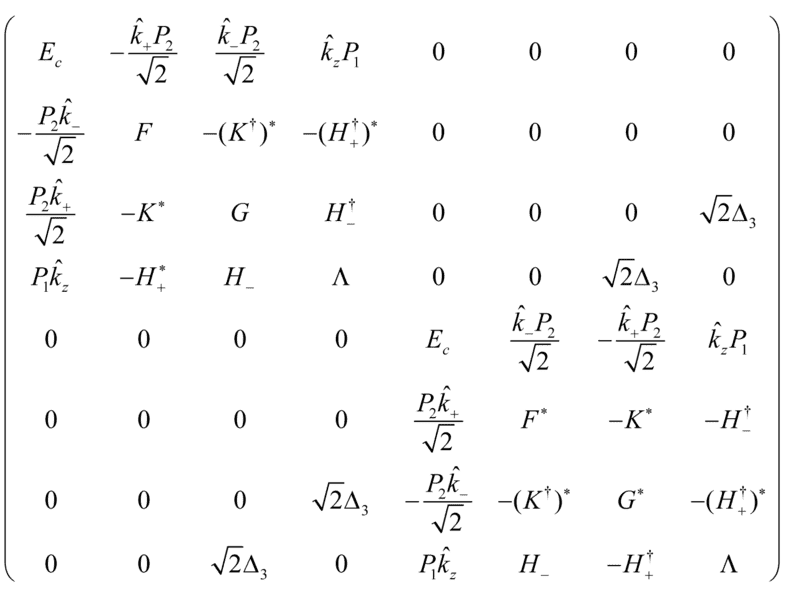# The hermicity of a k.p matrix?

• A
• lichen1983312

#### lichen1983312

I am trying to use the k.p method to study quantum well band structure. One example Hamiltonian look like this [J. Appl. Phys., 116, 033709(2014)]where
##{{\hat k}_ \pm } = {{\hat k}_x} \pm i{{\hat k}_y}##
and the matrix elements are function of ##{{\hat k}_i}##
and if quantum well is grown along z direction
the envelop functions have the form
$F(x,y,z) = {e^{i{{\bf{k}}_\parallel } \cdot {\bf{r}}}}f(z)$

and therefore

##\left\{ \begin{array}{l}
{{\hat k}_x} = - i\frac{\partial }{{\partial x}} \to {k_x}\\
{{\hat k}_y} = - i\frac{\partial }{{\partial y}} \to {k_y}\\
{{\hat k}_z} = - i\frac{\partial }{{\partial z}}
\end{array} \right.##

this Hamiltonian is an hermitian operator, but I am confused about this. For example, if I look at the (1,4) and (4,1) terms and use ${{\hat k}_z}=-i{\partial _z}$, the (1,4) term becomes
##-i{\partial _z}{P_1}##
and the (4,1) term becomes
##- i{P_1}{\partial _z}##

If I use finite difference method to turn these two terms into a matrix block, the factor -i is not going to flip sign and the matrix cannot he a hermitian matrix?

I have to look at the paper first, the matrix is hermitian, but I will say that $P_1$ and $P_2$ are constants, not operators if I remember my $\vec{k} \cdot \vec{p}$ theory correctly.
I have to look at the paper first, the matrix is hermitian, but I will say that $P_1$ and $P_2$ are constants, not operators if I remember my $\vec{k} \cdot \vec{p}$ theory correctly.